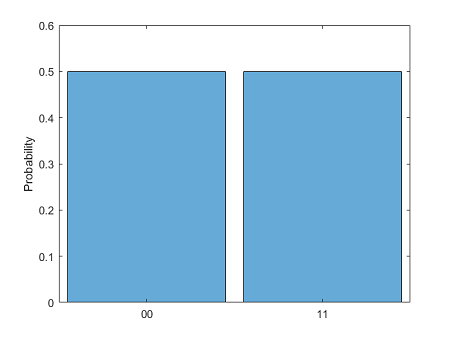# simulate

Simulate quantum circuit

Since R2023a

Installation Required: This functionality requires MATLAB Support Package for Quantum Computing.

## Syntax

``s = simulate(c)``
``s = simulate(c,inputState)``

## Description

example

````s = simulate(c)` simulates the quantum circuit `c` and returns the final state `s` after running the circuit. By default, each qubit in the circuit is initially in the $|0〉$ state.```

example

````s = simulate(c,inputState)` additionally specifies the initial quantum state of the circuit.The default value of `inputState` is `"0...0"` with as many `"0"` characters as there are qubits in `c`, where each qubit is in the $|0〉$ state. You can specify `inputState` as either a string containing `"0"`, `"1"`, `"+"`, or `"-"` with as many characters as there are qubits in `c` or a `QuantumState` object.```

## Examples

collapse all

Create a quantum circuit that consists of a Hadamard gate and a controlled X gate to entangle two qubits.

```gates = [hGate(1); cxGate(1,2)]; c = quantumCircuit(gates);```

Simulate the circuit using the default initial state where each qubit is in the $|0〉$ state.

`s = simulate(c)`
```s = QuantumState with properties: BasisStates: [4×1 string] Amplitudes: [4×1 double] NumQubits: 2```

Show the final state of the circuit.

`formula(s)`
```ans = "0.70711 * |00> + 0.70711 * |11>"```

Plot the histogram of probabilities to measure possible states from the final state of the circuit.

`histogram(s)`Create a quantum circuit that consists of a controlled X gate.

`c = quantumCircuit(cxGate(1,2));`

Simulate the circuit using initial states of `"00"`, `"01"`, `"10"`, and `"11"`. Show the final state of the circuit for each initial state after running the circuit.

```for ket=["00", "01", "10", "11"] s = simulate(c,ket); disp("|" + ket + "> -> " + formula(s)); end```
```|00> -> 1 * |00> |01> -> 1 * |01> |10> -> 1 * |11> |11> -> 1 * |10>```

## Input Arguments

collapse all

Quantum circuit, specified as a `quantumCircuit` object.

Initial state of quantum circuit, specified as a string scalar or a `QuantumState` object.

• The default value is `"0...0"` with as many characters `"0"` as there are qubits in `c`.

• You can specify a string containing `"0"`, `"1"`, `"+"`, or `"-"` with as many characters as there are qubits in `c`. For example, to specify four qubits that are in the initial state $|0+1-〉$, use `"0+1-"`.

• You can specify a `QuantumState` object. For example, you can construct the initial state $|0+1-〉$ using ```inputState = quantum.gate.QuantumState("0+1-")```. The `quantum.gate.QuantumState` constructor also accepts a complex vector to create a quantum state. For example, you can construct the initial state $\left(i|01〉+|10〉\right)/\sqrt{2}$ using ```inputState = quantum.gate.QuantumState([0 1i/sqrt(2) 1/sqrt(2) 0])```. You can also specify a `QuantumState` object that is returned by running `simulate` on another quantum circuit (with the same number of qubits).

## Output Arguments

collapse all

Final state after running the circuit, returned as a `QuantumState` object. This object contains the normalized amplitudes of all possible ${2}^{n}$ basis states of n qubits that form the quantum state. The quantum state can include a global phase or relative phases among the amplitudes of the basis states that do not affect the probabilities of measuring these states.

## Limitations

• Because the number of possible basis states of a quantum state scales as ${2}^{n}$ as the number of qubits n grows, using `simulate` is practical for finding the final state `s` of a circuit only when n is less than about 15.

## Version History

Introduced in R2023a